# 机器学习算法之线性判别分析(LDA)

LDA和PCA都是利用线性变换对数据进行降维的机器学习技术。之前也对主成分分析（PCA）原理进行了总结。PCA是一种无监督的降维技术，无视数据的分类信息挖掘数据中的模式，投影后方差最大的方向即为主成分。LDA是一种有监督的降维技术，对数据进行模式分类。如图所示，LDA要求类间的方差最大，而类内的方差最小，以保证投影后同一分类数据集中，不同分类间数据距离尽可能大。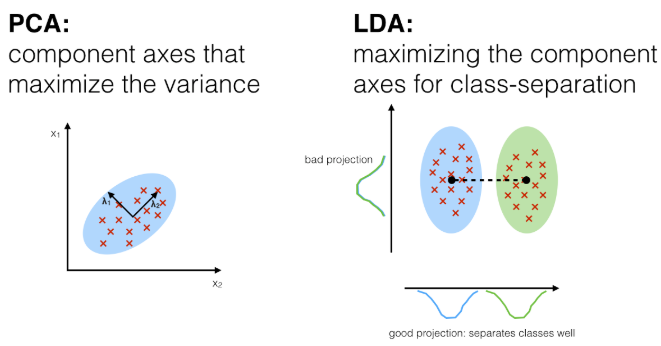LDA的思想可以用一句话概括，就是“投影后类内方差最小，类间方差最大”。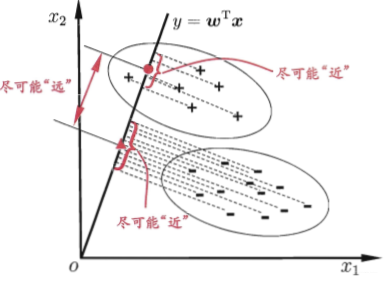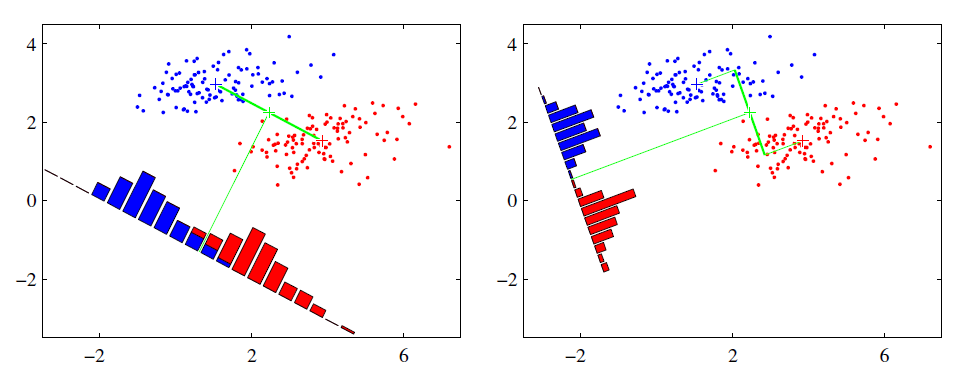## LDA原理推导

### 瑞利商（Rayleigh quotient）与广义瑞利商（genralized Rayleigh quotient）

$$R(A,x) = \frac{x^HAx}{x^Hx}$$

$$\lambda_{min} \leq \frac{x^HAx}{x^Hx} \leq \lambda_{max}$$

$$R(A,x) = \frac{x^HAx}{x^HBx}$$

$$x^HBx = x’^H(B^{-1/2})^HBB^{-1/2}x’ = x’^HB^{-1/2}BB^{-1/2}x’ = x’^Hx’$$

$$x^HAx = x’^HB^{-1/2}AB^{-1/2}x’$$

$$R(A,B,x’) = \frac{x’^HB^{-1/2}AB^{-1/2}x’}{x’^Hx’}$$

### 二类LDA原理

LDA希望投影后希望同一种类别数据的投影点尽可能的接近，而不同类别的数据的类别中心之间的距离尽可能的大，但是这只是一个感官的度量。现在我们首先从比较简单的二类LDA入手，严谨的分析LDA的原理。

$$\mu_j = \frac{1}{N_j}\sum\limits_{x \in X_j}x\;\;(j=0,1)$$

$$\Sigma_j = \sum\limits_{x \in X_j}(x-\mu_j)(x-\mu_j)^T\;\;(j=0,1)$$

$$\underbrace{arg\;max}_w\;\;J(w) = \frac{||w^T\mu_0-w^T\mu_1||_2^2}{w^T\Sigma_0w+w^T\Sigma_1w} = \frac{w^T(\mu_0-\mu_1)(\mu_0-\mu_1)^Tw}{w^T(\Sigma_0+\Sigma_1)w}$$

$$S_w = \Sigma_0 + \Sigma_1 = \sum\limits_{x \in X_0}(x-\mu_0)(x-\mu_0)^T + \sum\limits_{x \in X_1}(x-\mu_1)(x-\mu_1)^T$$

$$S_b = (\mu_0-\mu_1)(\mu_0-\mu_1)^T$$

$$\underbrace{arg\;max}_w\;\;J(w) = \frac{w^TS_bw}{w^TS_ww}$$

### 多类LDA原理

$$\frac{W^TS_bW}{W^TS_wW}$$

$$\underbrace{arg\;max}_W\;\;J(W) = \frac{\prod\limits_{diag}W^TS_bW}{\prod\limits_{diag}W^TS_wW}$$

$J(W)$的优化过程可以转化为：

$$J(W) = \frac{\prod\limits_{i=1}^dw_i^TS_bw_i}{\prod\limits_{i=1}^dw_i^TS_ww_i} = \prod\limits_{i=1}^d\frac{w_i^TS_bw_i}{w_i^TS_ww_i}$$

### LDA算法流程

LDA进行降维算法流程：

• 计算类内散度矩阵$S_w$
• 计算类间散度矩阵$S_b$
• 计算矩阵$S_w^{-1}S_b$
• 计算$S_w^{-1}S_b$的最大的d个特征值和对应的d个特征向量$(w_1,w_2,…w_d)$,得到投影矩阵W
• 对样本集中的每一个样本特征$x_i$，转化为新的样本$z_i=W^Tx_i$
• 得到输出样本集$D’=\{(z_1,y_1), (z_2,y_2), …,((z_m,y_m))\}$

## LDA算法小结

LDA用于降维，和PCA有很多相同，也有很多不同的地方，因此值得好好的比较一下两者的降维异同点。

• 两者均可以对数据进行降维
• 两者在降维时均使用了矩阵特征分解的思想
• 两者都假设数据符合高斯分布

• LDA是有监督的降维方法，而PCA是无监督的降维方法
• LDA降维最多降到类别数k-1的维数，而PCA没有这个限制
• LDA除了可以用于降维，还可以用于分类
• LDA选择分类性能最好的投影方向，而PCA选择样本点投影具有最大方差的方向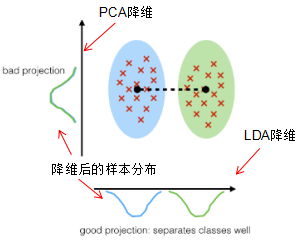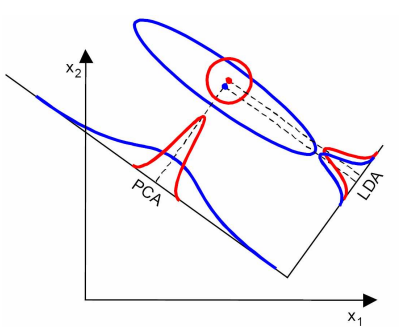LDA最多将数据降维至C-1维，也就是说如果有两类数据，最终降维只能到1维，也就是说投影到一个直线上。这在很多情况下无法对数据进行很好的投影，例如下图中的几种情况。也就是说，LDA不适合对非高斯分布的样本进行降维。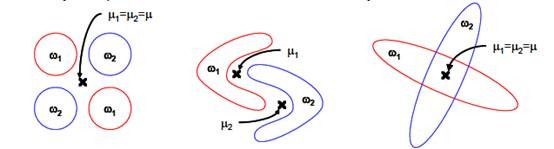• 在降维过程中可以使用类别的先验知识经验，而像PCA这样的无监督学习则无法使用类别先验知识。
• LDA在样本分类信息依赖均值而不是方差的时候，比PCA之类的算法较优。

• LDA不适合对非高斯分布样本进行降维，PCA也有这个问题
• LDA降维最多降到类别数k-1的维数，如果我们降维的维度大于k-1，则不能使用LDA。当然目前有一些LDA的进化版算法可以绕过这个问题
• LDA在样本分类信息依赖方差而不是均值的时候，降维效果不好
• LDA可能过度拟合数据

## LDA使用实例

sklearn实现：

class sklearn.discriminant_analysis.LinearDiscriminantAnalysis(solver='svd', shrinkage=None, priors=None, n_components=None, store_covariance=False, tol=0.0001)

• solver: 即求LDA超平面特征矩阵使用的方法。可以选择的方法有奇异值分解”svd”，最小二乘”lsqr”和特征分解”eigen”。一般来说特征数非常多的时候推荐使用svd，而特征数不多的时候推荐使用eigen。主要注意的是，如果使用svd，则不能指定正则化参数shrinkage进行正则化。默认值是svd
• shrinkage：正则化参数，可以增强LDA分类的泛化能力。如果仅仅只是为了降维，则一般可以忽略这个参数。默认是None，即不进行正则化。可以选择”auto”,让算法自己决定是否正则化。当然我们也可以选择不同的[0,1]之间的值进行交叉验证调参。注意shrinkage只在solver为最小二乘”lsqr”和特征分解”eigen”时有效。
• Shrinkage：str or float类型，默认值为None。是否使用参数收缩
• None：不使用参数收缩
• auto：str，使用Ledoit-Wolf lemma
• 浮点数：自定义收缩比例
• priors：类别权重，可以在做分类模型时指定不同类别的权重，进而影响分类模型建立。降维时一般不需要关注这个参数。
• n_components：即我们进行LDA降维时降到的维数。在降维时需要输入这个参数。注意只能为[1,类别数-1)范围之间的整数。如果我们不是用于降维，则这个值可以用默认的None。
• store_covariance：一个布尔值。如果为True，则需要额外计算每个类别的协方差矩阵。
• tol：一个浮点数。它指定了用于SVD算法中评判迭代收敛的阈值

import matplotlib.pyplot as plt
from sklearn import datasets
from sklearn.discriminant_analysis import LinearDiscriminantAnalysis

X = iris.data
y = iris.target
target_names = iris.target_names

lda = LinearDiscriminantAnalysis(n_components=2)
X_r2 = lda.fit(X, y).transform(X)

colors = ['navy', 'turquoise', 'darkorange']

for color, i, target_name in zip(colors, [0, 1, 2], target_names):
plt.scatter(X_r2[y == i, 0], X_r2[y == i, 1], alpha=.8, color=color,
label=target_name)
plt.title('LDA of IRIS dataset')

plt.show()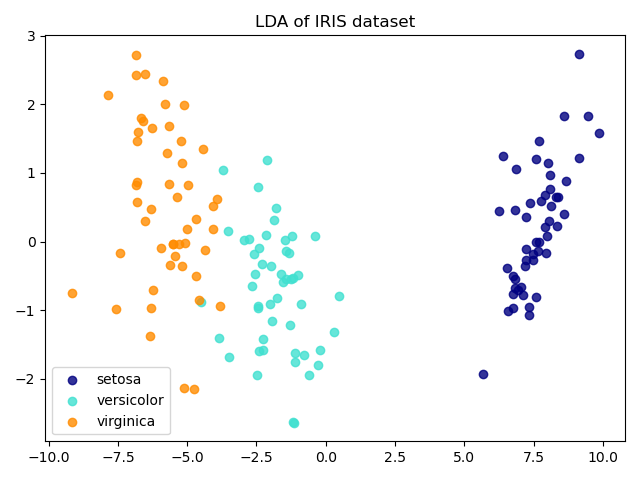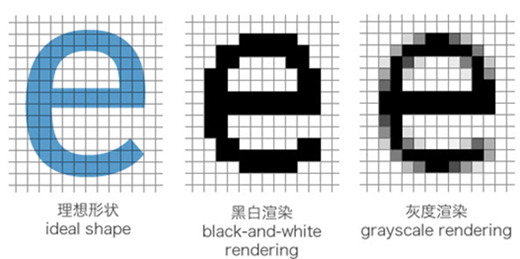##### 网站与APP开发中的字体设置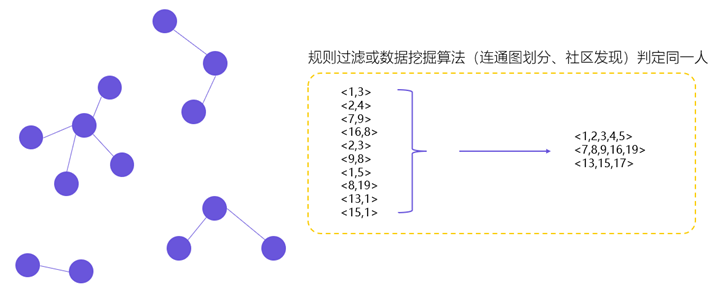##### 用户体系搭建之ID-Mapping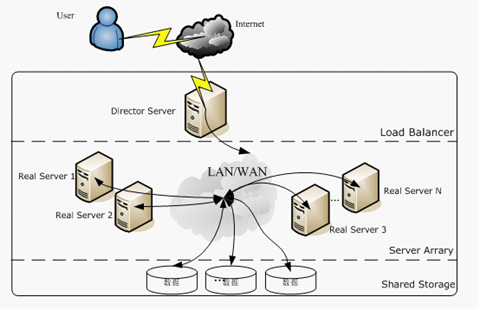## 2 Replies to “机器学习算法之线性判别分析(LDA)”

1. Jerry Mak说道：

写的很不错，但是第一句的linear错啦